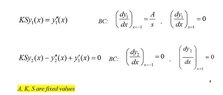# Help to find asymptotic solution of linear ode

• MHB
flanker
I have a trouble with ODE, I try to find asymptotic solution for odes which presented in pics. But I can’t. Please introduce a method which I solve these equations. I can solve these equations analytically but after solution, inverse Laplace transform must apply to find final answer. In analytical solution inverse Laplace transform near to impossible. Since I want a way to approximate analytical solution to these equations afterwards I can apply inverse Laplace transform to find final answer.
thanks
View attachment 5647

#### Attachments

•2s.jpg
12.8 KB · Views: 40

Homework Helper
MHB
I have a trouble with ODE, I try to find asymptotic solution for odes which presented in pics. But I can’t. Please introduce a method which I solve these equations. I can solve these equations analytically but after solution, inverse Laplace transform must apply to find final answer. In analytical solution inverse Laplace transform near to impossible. Since I want a way to approximate analytical solution to these equations afterwards I can apply inverse Laplace transform to find final answer.
thanks

Hi flanker! Welcome to MHB! ;)

Suppose $Y_1(s)$ is the Laplace transform of $y_1(x)$, then from the tables, we can see:
\begin{array}{lll}KSy_1 &= y_1'' & \text{Initial equation}\\
KS Y_1&= s^2 Y_1 - sy_1(0)-y_1'(0) & \text{Laplace transform} \\
Y_1 &= \frac{sy_1(0)+y_1'(0)}{s^2-KS} & \text{Solve for }Y_1 \\
Y_1 &= y_1(0)\frac{s}{s^2-KS} + y_1'(0)\frac{1}{s^2-KS} & \text{Prepare for Inverse Laplace transform}\\
y_1 &= y_1(0) \cosh(x\sqrt{KS}) + y_1'(0) \sinh(x\sqrt{KS}) & \text{Inverse Laplace transform}
\end{array}
Now we can fill in the boundary conditions to find $y_1(0)$ respectively $y_1'(0)$.

flanker
Thank you very much dear for your kindly attention to my question. but I applied a laplace transform to a PDE for x then these equation are producted. I must solve these equations and apply the inverse laplace transform to find final answer. But the initial answer of these equations are too complicated that I can't apply inverse laplace transform.:(

Homework Helper
MHB
Thank you very much dear for your kindly attention to my question. but I applied a laplace transform to a PDE for x then these equation are producted. I must solve these equations and apply the inverse laplace transform to find final answer. But the initial answer of these equations are too complicated that I can't apply inverse laplace transform.:(

There seems to be some misunderstanding.
It doesn't make sense to me to apply an inverse laplace transform to the solution of these ODE's.

Anyway, the inverse Laplace transform of for instance $\cosh(x\sqrt{KS})$ is $\frac 12\delta(t-\sqrt{KS}) + \frac 12\delta(t+\sqrt{KS})$.

Maybe you can provide the initial answer that an inverse laplace transform should be applied to?
And perhaps the original problem?

flanker
Dear sir can u give your mail which i want to send u an article

Homework Helper
MHB